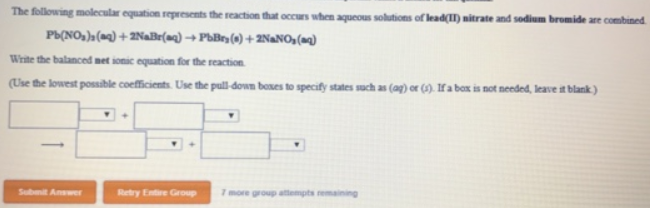# Problem: The following molecular equation represents the reaction that occurs when aqueous solutions of lead(lI) nitrate and sodium bromide are combined.Pb(NO3)2 (aq) + 2NaBr (aq) → PbBr3 (s) + 2NaNO3 (aq)Write the balanced net ionic equation for the reaction.(Use the lowest possible coefficents. Use the pull-down boxes to specify states such as (aq) or (l). If a box is not needed, leave it blank.)

###### FREE Expert Solution
81% (375 ratings)###### Problem Details

The following molecular equation represents the reaction that occurs when aqueous solutions of lead(lI) nitrate and sodium bromide are combined.

Pb(NO3)2 (aq) + 2NaBr (aq) → PbBr3 (s) + 2NaNO3 (aq)

Write the balanced net ionic equation for the reaction.

(Use the lowest possible coefficents. Use the pull-down boxes to specify states such as (aq) or (l). If a box is not needed, leave it blank.)